# 1 Tuesday, January 12

## 1.1 Background

From Phil’s email:

Personally, I found the following online references particularly useful:

• Dietmar Salamon: Spin Geometry and Seiberg-Witten Invariants 

• Richard Mandelbaum: Four-dimensional Topology: An Introduction 

• This book has a nice introduction to surgery aspects of four-manifolds, but as a warning: It was published right before Freedman’s famous theorem. For instance, the existence of an exotic R^4 was not known. This actually makes it quite useful, as a summary of what was known before, and provides the historical context in which Freedman’s theorem was proven.
• Danny Calegari: Notes on 4-Manifolds 

• Yuli Rudyak: Piecewise Linear Structures on Topological Manifolds 

• Akhil Mathew: The Dirac Operator 

• Tom Weston: An Introduction to Cobordism Theory 

A wide variety of lecture notes on the Atiyah-Singer index theorem, which are available online.

## 1.2 Introduction

Recall that a topological manifold (or $$C^0$$ manifold) $$X$$ is a Hausdorff topological space locally homeomorphic to $${\mathbb{R}}^n$$ with a countable topological base, so we have charts $$\phi_u: U\to {\mathbb{R}}^n$$ which are homeomorphisms from open sets covering $$X$$.

$$S^1$$ is covered by two charts homeomorphic to intervals: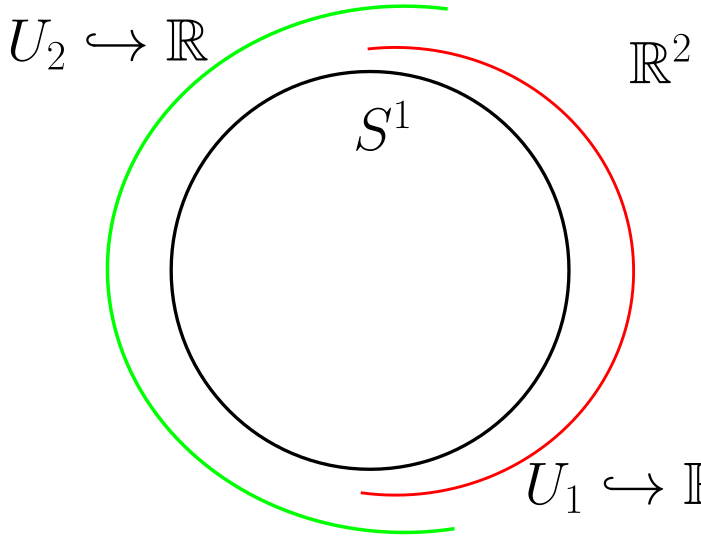Maps that are merely continuous are poorly behaved, so we may want to impose extra structure. This can be done by imposing restrictions on the transition functions, defined as \begin{align*} t_{uv} \mathrel{\vcenter{:}}=\varphi_V \to \varphi_U ^{-1} : \varphi_U(U \cap V) \to \varphi_V(U \cap V) .\end{align*}

• We say $$X$$ is a PL manifold if and only if $$t_{UV}$$ are piecewise-linear. Note that an invertible PL map has a PL inverse.

• We say $$X$$ is a $$C^k$$ manifold if they are $$k$$ times continuously differentiable, and smooth if infinitely differentiable.

• We say $$X$$ is real-analytic if they are locally given by convergent power series.

• We say $$X$$ is complex-analytic if under the identification $${\mathbb{R}}^n \cong {\mathbb{C}}^{n/2}$$ if they are holomorphic, i.e. the differential of $$t_{UV}$$ is complex linear.

• We say $$X$$ is a projective variety if it is the vanishing locus of homogeneous polynomials on $${\mathbb{CP}}^N$$.

Is this a strictly increasing hierarchy? It’s not clear e.g. that every $$C^k$$ manifold is PL.

Consider $${\mathbb{R}}^n$$ as a topological manifold: are any two smooth structures on $${\mathbb{R}}^n$$ diffeomorphic?

Fix a copy of $${\mathbb{R}}$$ and form a single chart $${\mathbb{R}}\xrightarrow{\operatorname{id}} {\mathbb{R}}$$. There is only a single transition function, the identity, which is smooth. But consider \begin{align*} X &\to {\mathbb{R}}\\ t &\mapsto t^3 .\end{align*} This is also a smooth structure on $$X$$, since the transition function is the identity. This yields a different smooth structure, since these two charts don’t like in the same maximal atlas. Otherwise there would be a transition function of the form $$t_{VU}: t\mapsto t^{1/3}$$, which is not smooth at zero. However, the map \begin{align*} X &\to X \\ t &\mapsto t^3 .\end{align*} defines a diffeomorphism between the two smooth structures.

$${\mathbb{R}}$$ admits a unique smooth structure.

Let $$\tilde {\mathbb{R}}$$ be some exotic $${\mathbb{R}}$$, i.e. a smooth manifold homeomorphic to $${\mathbb{R}}$$. Cover this by coordinate charts to the standard $${\mathbb{R}}$$: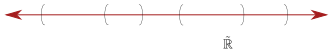There exists a cover which is locally finite and supports a partition of unity: a collection of smooth functions $$f_i: U_i \to {\mathbb{R}}$$ with $$f_i \geq 0$$ and $${\operatorname{supp}}f \subseteq U_i$$ such that $$\sum f_i = 1$$ (i.e., bump functions). It is also a purely topological fact that $$\tilde {\mathbb{R}}$$ is orientable.

So we have bump functions: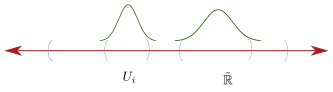Take a smooth vector field $$V_i$$ on $$U_i$$ everywhere aligning with the orientation. Then $$\sum f_i V_i$$ is a smooth nowhere vector field on $$X$$ that is nowhere zero in the direction of the orientation. Taking the associated flow \begin{align*} {\mathbb{R}}&\to \tilde {\mathbb{R}}\\ t &\mapsto \varphi(t) .\end{align*} such that $$\varphi'(t) = V(\varphi(t))$$. Then $$\varphi$$ is a smooth map that defines a diffeomorphism. This follows from the fact that the vector field is everywhere positive.

To understand smooth structures on $$X$$, we should try to solve differential equations on $$X$$.

Note that here we used the existence of a global frame, i.e. a trivialization of the tangent bundle, so this doesn’t quite work for e.g. $$S^2$$.

What is the difference between all of the above structures? Are there obstructions to admitting any particular one?

1. (Munkres) Every $$C^1$$ structure gives a unique $$C^k$$ and $$C^ \infty$$ structure.1

2. (Grauert) Every $$C^ \infty$$ structure gives a unique real-analytic structure.

3. Every PL manifold admits a smooth structure in $$\dim X \leq 7$$, and it’s unique in $$\dim X\leq 6$$, and above these dimensions there exists PL manifolds with no smooth structure.

4. (Kirby–Siebenmann) Let $$X$$ be a topological manifold of $$\dim X\geq 5$$, then there exists a cohomology class $$\operatorname{ks}(X) \in H^4(X; {\mathbb{Z}}/2{\mathbb{Z}})$$ which is 0 if and only if $$X$$ admits a PL structure. Moreover, if $$\operatorname{ks}(X) = 0$$, then (up to concordance) the set of PL structures is given by $$H^3(X; {\mathbb{Z}}/2{\mathbb{Z}})$$.

5. (Moise) Every topological manifold in $$\dim X\leq 3$$ admits a unique smooth structure.

6. (Smale et al.): In $$\dim X\geq 5$$, the number of smooth structures on a topological manifold $$X$$ is finite. In particular, $${\mathbb{R}}^n$$ for $$n \neq 4$$ has a unique smooth structure. So dimension 4 is interesting!

7. (Taubes) $${\mathbb{R}}^4$$ admits uncountably many non-diffeomorphic smooth structures.

8. A compact oriented smooth surface $$\Sigma$$, the space of complex-analytic structures is a complex orbifold2 of dimension $$3g-2$$ where $$g$$ is the genus of $$\Sigma$$, up to biholomorphism (i.e. moduli).

Kervaire-Milnor: $$S^7$$ admits 28 smooth structures, which form a group.

# 2 Friday, January 15

Let \begin{align*} V &\mathrel{\vcenter{:}}=\left\{{a^2 + b^2 + c^2 + d^3 + e^{6k-1} = 0}\right\} \subseteq {\mathbb{C}}^5 \\ S_{\varepsilon}&\mathrel{\vcenter{:}}=\left\{{ {\left\lvert {a} \right\rvert}^2 + {\left\lvert {b} \right\rvert}^2 + {\left\lvert {c} \right\rvert}^2 + {\left\lvert {d} \right\rvert}^2 + {\left\lvert {e} \right\rvert}^2 = 1}\right\} .\end{align*} Then $$V_k \cap S_{\varepsilon}\cong S^7$$ is a homeomorphism, and taking $$k=1,2,\cdots, 28$$ yields the 28 smooth structures on $$S^7$$. Note that $$V_k$$ is the cone over $$V_k \cap S_{\varepsilon}$$.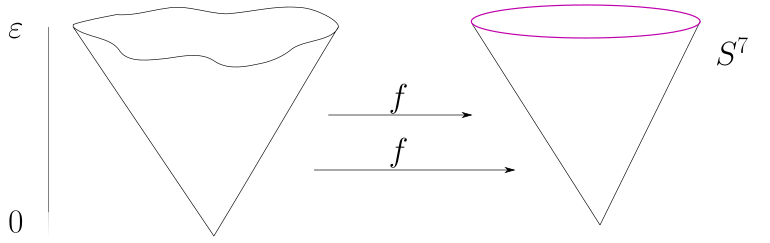? Admits a smooth structure, and $$\mkern 1.5mu\overline{\mkern-1.5muV\mkern-1.5mu}\mkern 1.5mu_k \subseteq {\mathbb{CP}}^5$$ admits no smooth structure.

Is every triangulable manifold PL, i.e. homeomorphic to a simplicial complex?

No! Given a simplicial complex, there is a notion of the combinatorial link $$L_V$$ of a vertex $$V$$: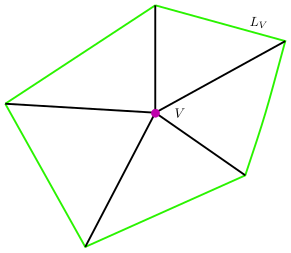It turns out that there exist simplicial manifolds such that the link is not homeomorphic to a sphere, whereas every PL manifold admits a “PL triangulation” where the links are spheres.

What’s special in dimension 4? Recall the Kirby-Siebenmann invariant $$\operatorname{ks}(x) \in H^4(X; {\mathbb{Z}}_2)$$ for $$X$$ a topological manifold where $$\operatorname{ks}(X) = 0 \iff X$$ admits a PL structure, with the caveat that $$\dim X \geq 5$$. We can use this to cook up an invariant of 4-manifolds.

Let $$X$$ be a topological 4-manifold, then \begin{align*} \operatorname{ks}(X) \mathrel{\vcenter{:}}=\operatorname{ks}(X \times{\mathbb{R}}) .\end{align*}

Recall that in $$\dim X\geq 7$$, every PL manifold admits a smooth structure, and we can note that \begin{align*} H^4(X; {\mathbb{Z}}_2) = H^4(X \times{\mathbb{R}}; {\mathbb{Z}}_2) = {\mathbb{Z}}_2, .\end{align*} since every oriented 4-manifold admits a fundamental class. Thus \begin{align*} \operatorname{ks}(X) = \begin{cases} 0 & X \times{\mathbb{R}}\text{ admits a PL and smooth structure} \\ 1 & X \times{\mathbb{R}}\text{ admits no PL or smooth structures }. \end{cases} \end{align*}

$$\operatorname{ks}(X) \neq 0$$ implies that $$X$$ has no smooth structure, since $$X \times{\mathbb{R}}$$ doesn’t. Note that it was not known if this invariant was ever nonzero for a while!

Note that $$H^2(X; {\mathbb{Z}})$$ admits a symmetric bilinear form $$Q_X$$ defined by \begin{align*} Q_X: H^2(X; {\mathbb{Z}})^{\otimes 2} &\to {\mathbb{Z}}\\ \alpha \otimes\beta &\mapsto \int_X \alpha\wedge \beta \mathrel{\vcenter{:}}=(\alpha \smile\beta)([X]) .\end{align*} where $$[X]$$ is the fundamental class.

# 3 Main Theorems for the Course

Proving the following theorems is the main goal of this course:

If $$X, Y$$ are compact oriented topological 4-manifolds, then $$X\cong Y$$ are homeomorphic if and only if $$\operatorname{ks}(X) = \operatorname{ks}(Y)$$ and $$Q_X \cong Q_Y$$ are isometric, i.e. there exists an isometry \begin{align*} \varphi: H^2(X; {\mathbb{Z}}) \to H^2(Y; {\mathbb{Z}}) .\end{align*} that preserves the two bilinear forms in the sense that $${\left\langle {\varphi \alpha},~{ \varphi \beta} \right\rangle} = {\left\langle { \alpha},~{ \beta} \right\rangle}$$.

Conversely, every unimodular bilinear form appears as $$H^2(X; {\mathbb{Z}})$$ for some $$X$$, i.e. the pairing induces a map \begin{align*} H^2(X; {\mathbb{Z}}) &\to H^2(X; {\mathbb{Z}}) {}^{ \vee }\\ \alpha &\mapsto {\left\langle { \alpha },~{ {-}} \right\rangle} .\end{align*} which is an isomorphism. This is essentially a classification of simply-connected 4-manifolds.

Note that preservation of a bilinear form is a stand-in for “being an element of the orthogonal group,” where we only have a lattice instead of a full vector space.

There is a map $$H^2(X; {\mathbb{Z}}) \xrightarrow{PD} H_2(X; {\mathbb{Z}})$$ from Poincaré , where we can think of elements in the latter as closed surfaces $$[\Sigma]$$, and \begin{align*} {\left\langle { \Sigma_1 },~{ \Sigma_2 } \right\rangle} = \text{signed number of intersections points of } \Sigma_1 \pitchfork\Sigma_2 .\end{align*} Note that Freedman’s theorem is only about homeomorphism, and is not true smoothly. This gives a way to show that two 4-manifolds are homeomorphic, but this is hard to prove! So we’ll black-box this, and focus on ways to show that two smooth 4-manifolds are not diffeomorphic, since we want homeomorphic but non-diffeomorphic manifolds.

The signature of a topological 4- manifold is the signature of $$Q_X$$, where we note that $$Q_X$$ is a symmetric nondegenerate bilinear form on $$H^2(X; {\mathbb{R}})$$ and for some $$a, b$$ \begin{align*} (H^2(X; {\mathbb{R}}), Q_x) \xrightarrow{\text{isometric}} {\mathbb{R}}^{a, b} .\end{align*} where $$a$$ is the number of $$+1$$s appearing in the matrix and $$b$$ is the number of $$-1$$s. This is $${\mathbb{R}}^{ab}$$ where $$e_i^2 = 1, i=1\cdots a$$ and $$e_i^2 = -1, i=a+1, \cdots b$$, and is thus equipped with a specific bilinear form corresponding to the Gram matrix of this basis. \begin{align*} \begin{bmatrix} 1 & 0 & 0 & 0 & 0 \\ 0 & 1 & 0 & 0 & 0 \\ 0 & 0 & \ddots & 0 & 0 \\ 0 & 0 & 0 & -1 & 0 \\ 0 & 0 & 0 & 0 & -1 \end{bmatrix} = I_{a\times a} \oplus -I_{b \times b} .\end{align*} Then the signature is $$a-b$$, the dimension of the positive-definite space minus the dimension of the negative-definite space.

Suppose $${\left\langle { \alpha},~{\alpha} \right\rangle} \in 2{\mathbb{Z}}$$ and $$\alpha\in H^2(X; {\mathbb{Z}})$$ and $$X$$ a simply connected smooth 4-manifold. Then 16 divides $$\operatorname{sig}(X)$$.

Note that Freedman’s theorem implies that there exists topological 4-manifolds with no smooth structure.

Let $$X$$ be a smooth simply-connected 4-manifold. If $$a=0$$ or $$b=0$$, then $$Q_X$$ is diagonalizable and there exists an orthonormal basis of $$H^2(X; {\mathbb{Z}})$$.

This comes from Gram-Schmidt, and restricts what types of intersection forms can occur.

## 3.1 Warm Up: $${\mathbb{R}}^2$$ Has a Unique Smooth Structure

Last time we showed $${\mathbb{R}}^1$$ had a unique smooth structure, so now we’ll do this for $${\mathbb{R}}^2$$. The strategy of solving a differential equation, we’ll now sketch the proof.

A Riemannian metric $$g\in \Gamma( \operatorname{Sym}^2 T {}^{ \vee }X)$$ for $$X$$ a smooth manifold is a metric on every $$T_p X$$, so $$g_p \in (T_p X^{\otimes 2}) {}^{ \vee }$$, such that \begin{align*} g_p: T_pX \otimes T_p X &\to {\mathbb{R}}&& g(v, v) \geq 0, \quad g(v,v) = 0 \iff v=0 .\end{align*}

An almost complex structure is a morphism $$J\in \mathop{\mathrm{End}}_{{ \mathsf{Vect} }(X)}(TX)$$ of vector bundles over $$X$$ such that $$J^2 = -\operatorname{id}_{TX}$$.

An almost-complex structure is integrable $$J$$ if it comes from a complex structure in the following sense: for a complex manifold $$M\in {\mathsf{Mfd}}({\mathbb{C}})$$, take holomorphic coordinates $$z = x+iy$$ and set $$J {\frac{\partial }{\partial x}\,} \mathrel{\vcenter{:}}={\frac{\partial }{\partial y}\,}$$ and $$J{\frac{\partial }{\partial y}\,} \mathrel{\vcenter{:}}=-{\frac{\partial }{\partial x}\,}$$.

A manifold $$M\in {\mathsf{sm}}{\mathsf{Mfd}}({\mathbb{R}})$$ admits an almost-complex structure iff $$TM$$ admits a reduction of structure group $$\operatorname{GL}_{2n}({\mathbb{R}}) \to \operatorname{GL}_n({\mathbb{C}})$$.

Let $$e\in T_p X$$ and $$e\neq 0$$, then if $$X$$ is a surface then $$\left\{{e, J_p e}\right\}$$ is a basis of $$T_p X$$, where $$J_p$$ is the restriction of $$J$$ to $$T_p X$$: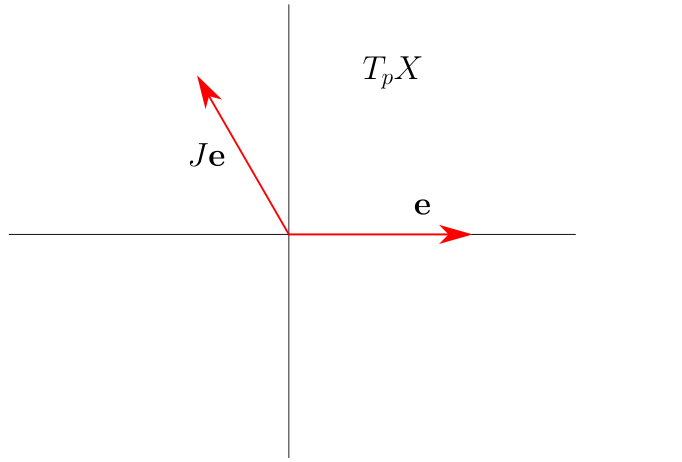Show that $$\left\{{ e, J_p e }\right\}$$ are linearly independent in $$T_p X$$. In particular, $$J_p$$ is determined by a point in $${\mathbb{R}}^2\setminus\left\{{\text{the }x{\hbox{-}}\text{axis}}\right\}$$

Let $$\tilde {\mathbb{R}}^2$$ be an exotic $${\mathbb{R}}^2$$.

### 3.1.1 Step 1

Choose a metric on $$\tilde {\mathbb{R}}^2$$, say $$g \mathrel{\vcenter{:}}=\sum f_I g_i$$ with $$g_i$$ metrics on coordinate charts $$U_i$$ and $$f_i$$ a partition of unity.

### 3.1.2 Step 2

Find an almost complex structure on $$\tilde {\mathbb{R}}^2$$. Choosing an orientation of $$\tilde {\mathbb{R}}^2$$, the metric $$g$$ defines a unique almost complex structure $$J_p e \mathrel{\vcenter{:}}= f\in T_p \tilde {\mathbb{R}}^2$$ such that

• $$g(e, e) = g(f, f)$$
• $$g(e, f) = 0$$.
• $$\left\{{e, f}\right\}$$ is an oriented basis of $$T_p \tilde {\mathbb{R}}^2$$

This is because after choosing $$e$$, there are two orthogonal vectors, but only one choice yields an oriented basis.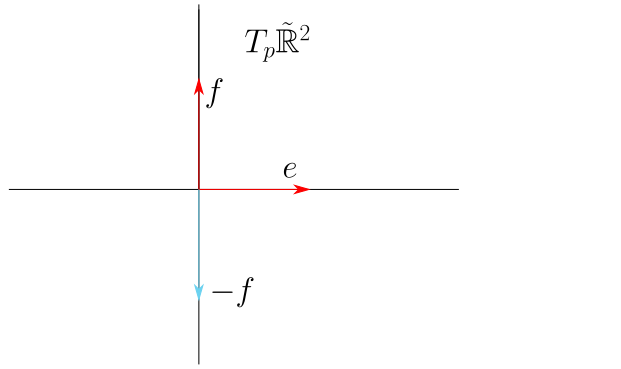### 3.1.3 Step 3

We then apply a theorem:

Any almost complex structure on a surface comes from a complex structure, in the sense that there exist charts $$\varphi_i: U_i \to {\mathbb{C}}$$ such that $$J$$ is multiplication by $$i$$.

So \begin{align*} d \varphi(J \cdot e) = i \cdot d \varphi_i (e) ,\end{align*} and $$(\tilde {\mathbb{R}}^2, J)$$ is a complex manifold. Since it’s simply connected, the Riemann Mapping Theorem shows that it’s biholomorphic to $${\mathbb{D}}$$ or $${\mathbb{C}}$$, both of which are diffeomorphic to $${\mathbb{R}}^2$$.

See the Newlander-Nirenberg theorem, a result in complex geometry.

# 4 Sheaves, Bundles, Connections (Lecture 3, Wednesday, January 20)

## 4.1 Sheaves

Recall that if $$X$$ is a topological space, a presheaf of abelian groups $$\mathcal{F}$$ is an assignment $$U\to \mathcal{F}(U)$$ of an abelian group to every open set $$U \subseteq X$$ together with a restriction map $$\rho_{UV}: \mathcal{F}(U) \to \mathcal{F}(V)$$ for any inclusion $$V \subseteq U$$ of open sets. This data has to satisfying certain conditions:

1. $$\mathcal{F}(\emptyset) = 0$$, the trivial abelian group.

2. $$\rho_{UU}: \mathcal{F}(U) \to \mathcal{F}(U) = \operatorname{id}_{\mathcal{F}(U) }$$

3. Compatibility if restriction is taken in steps: $$U \subseteq V \subseteq W \implies \rho_{VW} \circ \rho_{UV} = \rho_{UW}$$.

We say $$\mathcal{F}$$ is a sheaf if additionally:

1. Given $$s_i \in \mathcal{F}(U_i)$$ such that $$\rho_{U_i \cap U_j} (s_i) = \rho_{U_i \cap U_j} (s_j)$$ implies that there exists a unique $$s\in \mathcal{F}\qty{\displaystyle\bigcup_i U_i}$$ such that $$\rho_{U_i}(s) = s_i$$.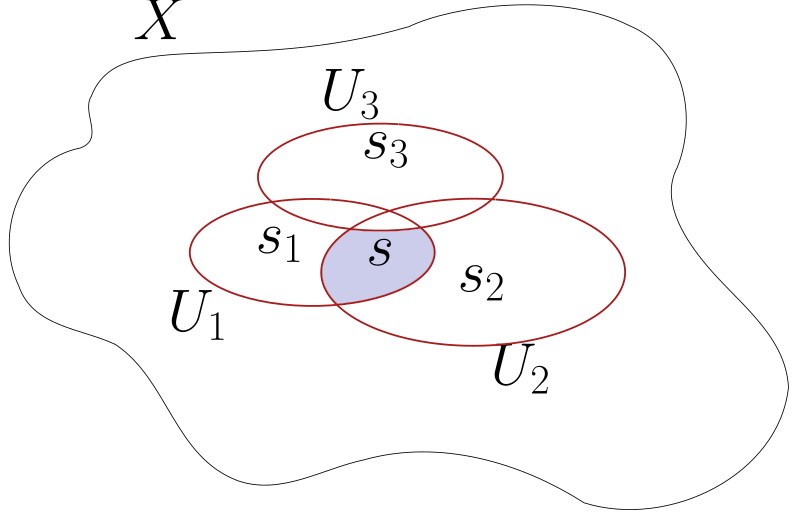Let $$X$$ be a topological manifold, then $$\mathcal{F}\mathrel{\vcenter{:}}= C^0({-}, {\mathbb{R}})$$ the set of continuous functionals form a sheaf. We have a diagramProperty (d) holds because given sections $$s_i \in C^0(U_i; {\mathbb{R}})$$ agreeing on overlaps, so $${ \left.{{s_i}} \right|_{{U_i \cap U_j}} } = { \left.{{s_j}} \right|_{{U_i \cap U_j}} }$$, there exists a unique $$s\in C^0\qty{\displaystyle\bigcup_i U_i; {\mathbb{R}}}$$ such that $${ \left.{{s}} \right|_{{U_i}} } = s_i$$ for all $$i$$ – i.e. continuous functions glue.

Recall that we discussed various structures on manifolds: PL, continuous, smooth, complex-analytic, etc. We can characterize these by their sheaves of functions, which we’ll denote $${\mathcal{O}}$$. For example, $${\mathcal{O}}\mathrel{\vcenter{:}}= C^0({-}; {\mathbb{R}})$$ for topological manifolds, and $${\mathcal{O}}\mathrel{\vcenter{:}}= C^ \infty ({-}; {\mathbb{R}})$$ is the sheaf for smooth manifolds. Note that this also works for PL functions, since pullbacks of PL functions are again PL. For complex manifolds, we set $${\mathcal{O}}$$ to be the sheaf of holomorphic functions.

Let $$A\in {\mathsf{Ab}}$$ be an abelian group, then $$\underline{A}$$ is the sheaf defined by setting $$\underline{A}(U)$$ to be the locally constant functions $$U\to A$$. E.g. let $$X \in {\mathsf{Mfd}}_{{\mathsf{Top}}}$$ be a topological manifold, then $$\underline{{\mathbb{R}}}(U) = {\mathbb{R}}$$ if $$U$$ is connected since locally constant $$\implies$$ globally constant in this case.

Note that the presheaf of constant functions doesn’t satisfy (d)! Take $${\mathbb{R}}$$ and a function with two different values on disjoint intervals: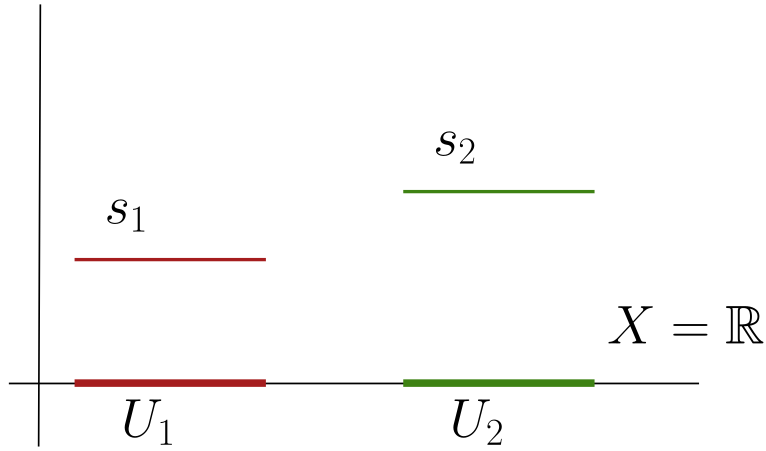Note that $${ \left.{{s_1}} \right|_{{U_1 \cap U_2}} } = { \left.{{s_2}} \right|_{{U_1 \cap U_2}} }$$ since the intersection is empty, but there is no constant function that restricts to the two different values.

## 4.2 Bundles

Let $$\pi: \mathcal{E}\to X$$ be a vector bundle, so we have local trivializations $$\pi ^{-1} (U) \xrightarrow{h_u} Y^d \times U$$ where we take either $$Y={\mathbb{R}}, {\mathbb{C}}$$, such that $$h_v \circ h_u ^{-1}$$ preserves the fibers of $$\pi$$ and acts linearly on each fiber of $$Y\times(U \cap V)$$. Define \begin{align*} t_{UV}: U \cap V \to \operatorname{GL}_d(Y) \end{align*} where we require that $$t_{UV}$$ is continuous, smooth, complex-analytic, etc depending on the context.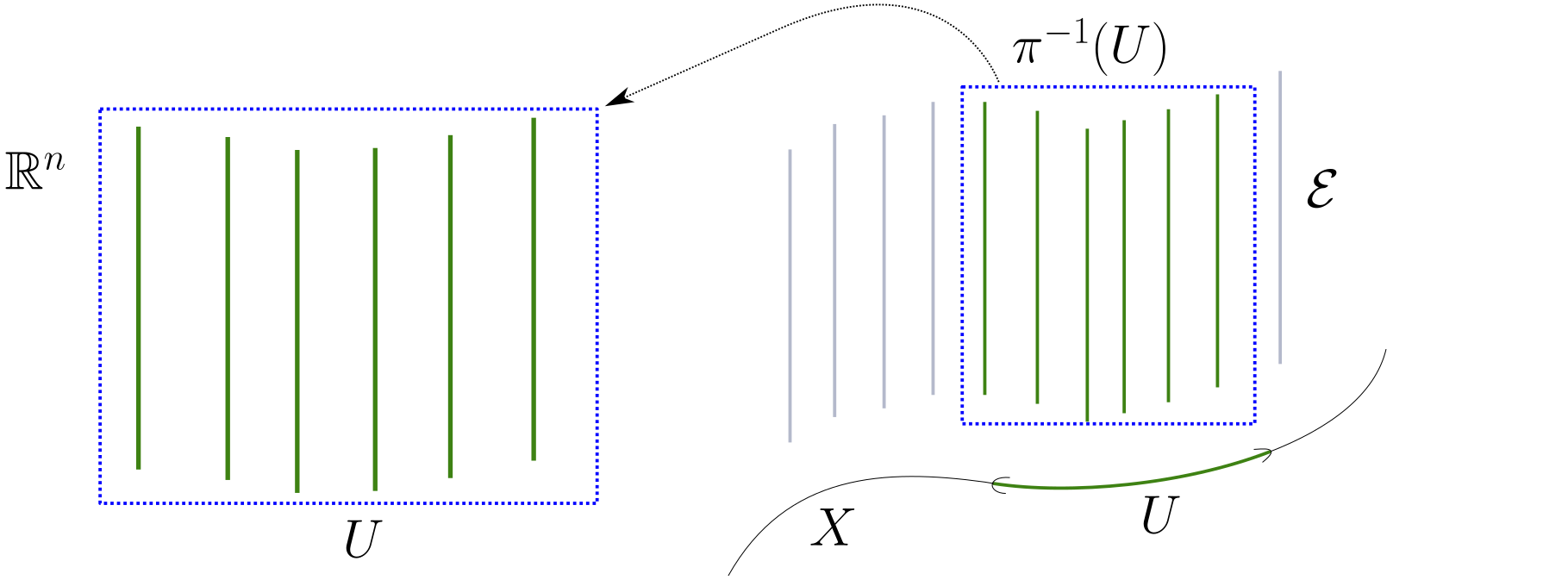There are two $${\mathbb{R}}^1$$ bundles over $$S^1$$:Note that the Mobius bundle is not trivial, but can be locally trivialized.

We abuse notation: $$\mathcal{E}$$ is also a sheaf, and we write $$\mathcal{E}(U)$$ to be the set of sections $$s: U\to \mathcal{E}$$ where $$s$$ is continuous, smooth, holomorphic, etc where $$\pi \circ s = \operatorname{id}_U$$. I.e. a bundle is a sheaf in the sense that its sections form a sheaf.

The trivial line bundle gives the sheaf $${\mathcal{O}}$$ : maps $$U \xrightarrow{s} U\times Y$$ for $$Y={\mathbb{R}}, {\mathbb{C}}$$ such that $$\pi \circ s = \operatorname{id}$$ are the same as maps $$U\to Y$$.

An $${\mathcal{O}}{\hbox{-}}$$module is a sheaf $$\mathcal{F}$$ such that $$\mathcal{F}(U)$$ has an action of $$\mathcal{O}(U)$$ compatible with restriction.

If $$\mathcal{E}$$ is a vector bundle, then $$\mathcal{E}(U)$$ has a natural action of $${\mathcal{O}}(U)$$ given by $$f\curvearrowright s \mathrel{\vcenter{:}}= fs$$, i.e. just multiplying functions.

The locally constant sheaf $$\underline{{\mathbb{R}}}$$ is not an $${\mathcal{O}}{\hbox{-}}$$module: there isn’t natural action since the sections of $${\mathcal{O}}$$ are generally non-constant functions, and multiplying a constant function by a non-constant function doesn’t generally give back a constant function.

We’d like a notion of maps between sheaves:

A morphism of sheaves $$\mathcal{F} \to \mathcal{G}$$ is a group morphism $$\varphi(U): \mathcal{F}(U) \to \mathcal{G}(U)$$ for all opens $$U \subseteq X$$ such that the diagram involving restrictions commutes:Let $$X = {\mathbb{R}}$$ and define the skyscraper sheaf at $$p \in {\mathbb{R}}$$ as \begin{align*} {\mathbb{R}}_p(U) \mathrel{\vcenter{:}}= \begin{cases} {\mathbb{R}}& p\in U \\ 0 & p\not\in U. \end{cases} .\end{align*}

The $${\mathcal{O}}(U){\hbox{-}}$$module structure is given by \begin{align*} {\mathcal{O}}(U) \times{\mathcal{O}}(U) &\to {\mathbb{R}}_p(U) \\ (f, s) &\mapsto f(p) s .\end{align*} This is not a vector bundle since $${\mathbb{R}}_p(U)$$ is not an infinite dimensional vector space, whereas the space of sections of a vector bundle is generally infinite dimensional (?). Alternatively, there are arbitrarily small punctured open neighborhoods of $$p$$ for which the sheaf makes trivial assignments.

Let $$X = {\mathbb{R}}\in {\mathsf{sm}}{\mathsf{Mfd}}$$ viewed as a smooth manifold, then multiplication by $$x$$ induces a morphism of structure sheaves: \begin{align*} (x \cdot): {\mathcal{O}}&\to {\mathcal{O}}\\ s & \mapsto x\cdot s \end{align*} for any $$x\in {\mathcal{O}}(U)$$, noting that $$x\cdot s\in {\mathcal{O}}(U)$$ again.

Check that $$\ker \varphi$$ is naturally a sheaf and $$\ker(\varphi)(U) = \ker (\varphi(U)): \mathcal{F}(U) \to \mathcal{G}(U)$$

Here the kernel is trivial, i.e. on any open $$U$$ we have $$(x\cdot):{\mathcal{O}}(U) \hookrightarrow{\mathcal{O}}(U)$$ is injective. Taking the cokernel $$\operatorname{coker}(x\cdot)$$ as a presheaf, this assigns to $$U$$ the quotient presheaf $${\mathcal{O}}(U) / x{\mathcal{O}}(U)$$, which turns out to be equal to $${\mathbb{R}}_0$$. So $${\mathcal{O}}\to {\mathbb{R}}_0$$ by restricting to the value at $$0$$, and there is an exact sequence \begin{align*} 0 \to {\mathcal{O}}\xrightarrow{(x\cdot)} {\mathcal{O}}\to {\mathbb{R}}_0 \to 0 .\end{align*}

This is one reason sheaves are better than vector bundles: the category is closed under taking quotients, whereas quotients of vector bundles may not be vector bundles.

# 5 Lecture 4 (Friday, January 22)

## 5.1 The Exponential Exact Sequence

Let $$X = {\mathbb{C}}$$ and consider $${\mathcal{O}}$$ the sheaf of holomorphic functions and $${\mathcal{O}}^{\times}$$ the sheaf of nonvanishing holomorphic functions. The former is a vector bundle and the latter is a sheaf of abelian groups. There is a map $$\exp: {\mathcal{O}}\to {\mathcal{O}}^{\times}$$, the exponential map, which is the data $$\exp(U): {\mathcal{O}}(U) \to {\mathcal{O}}^{\times}(U)$$ on every open $$U$$ given by $$f\mapsto e^f$$. There is a kernel sheaf $$2\pi i \underline{{\mathbb{Z}}}$$, and we get an exact sequence \begin{align*} 0 \to 2\pi i \underline{{\mathbb{Z}}} \to {\mathcal{O}}\xrightarrow{\exp} {\mathcal{O}}^{\times}\to \operatorname{coker}(\exp) \to 0 .\end{align*}

What is the cokernel sheaf here?

Let $$U$$ be a contractible open set, then we can identify $${\mathcal{O}}^{\times}(U) / \exp({\mathcal{O}}^{\times}(U)) = 1$$.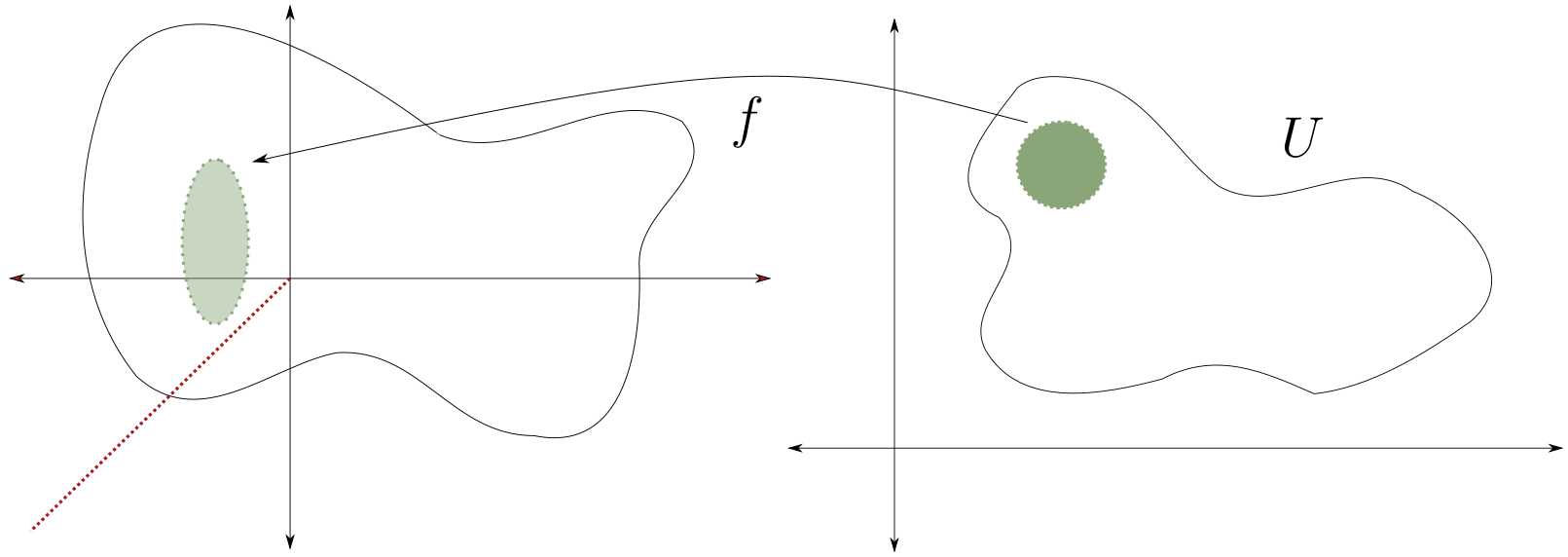Any $$f\in {\mathcal{O}}^{\times}(U)$$ has a logarithm, say by taking a branch cut, since $$\pi_1(U) =0 \implies \log f$$ has an analytic continuation. Consider the annulus $$U$$ and the function $$z\in {\mathcal{O}}^{\times}(U)$$, then $$z\not\in \exp({\mathcal{O}}(U))$$ – if $$z=e^f$$ then $$f=\log(z)$$, but $$\log(z)$$ has monodromy on $$U$$: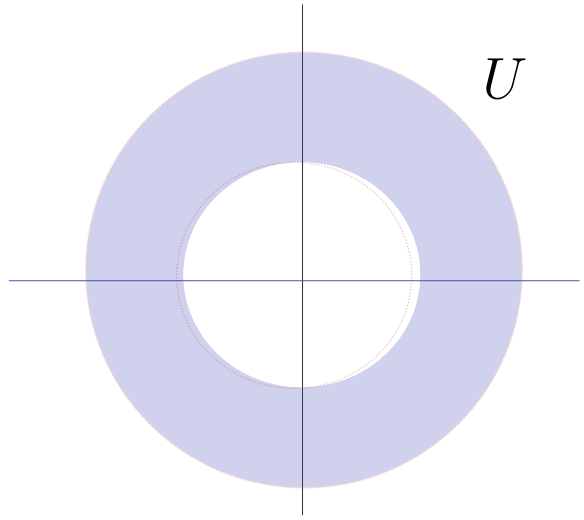Thus on any sufficiently small open set, $$\operatorname{coker}(\exp) = 1$$. This is only a presheaf: there exists an open cover of the annulus for which $${ \left.{{z}} \right|_{{U_i}} }$$, and so the naive cokernel doesn’t define a sheaf. This is because we have a locally trivial section which glues to $$z$$, which is nontrivial.

Redefine the cokernel so that it is a sheaf. Hint: look at sheafification, which has the defining property \begin{align*} \mathop{\mathrm{Hom}}_{ \underset{ \mathsf{pre} } {\mathsf{Sh} }}(\mathcal{G}, \mathcal{F}^{ \underset{ \mathsf{pre} } {\mathsf{Sh} }} ) =\mathop{\mathrm{Hom}}_{{\mathsf{Sh}}}( \mathcal{G}, \mathcal{F}^{{\mathsf{Sh}}}) \end{align*} for any sheaf $$\mathcal{G}$$.

## 5.2 Global Sections

The global sections sheaf of $$\mathcal{F}$$ on $$X$$ is given by $$H^0( X; \mathcal{F}) = \mathcal{F}(X)$$.

• $$C^ \infty (X) = H^0(X, C^ \infty )$$ are the smooth functions on $$X$$
• $$VF(X) = H^0(X; T)$$ are the smooth vector fields on $$X$$ for $$T$$ the tangent bundle
• If $$X$$ is a complex manifold then $${\mathcal{O}}(X) = H^0(X; {\mathcal{O}})$$ are the globally holomorphic functions on $$X$$.
• $$H^0(X; {\mathbb{Z}}) = \underline{{\mathbb{Z}}}(X)$$ are ??

Given vector bundles $$V, W$$, we have constructions $$V \oplus W, V \otimes W, V {}^{ \vee }, \mathop{\mathrm{Hom}}(V, W) = V {}^{ \vee }\otimes W, \operatorname{Sym}^n V, \bigwedge^p V$$, and so on. Some of these work directly for sheaves:

• $$\mathcal{F} \oplus \mathcal{G}(U) \mathrel{\vcenter{:}}=\mathcal{F}(U) \oplus \mathcal{G}(U)$$
• For tensors, duals, and homs $$\mathscr{H}\kern-2pt\operatorname{om}(V, W)$$ we only get presheaves, so we need to sheafify.

$$\mathop{\mathrm{Hom}}(V, W)$$ will denote the global homomorphisms $$\mathscr{H}\kern-2pt\operatorname{om}(V, W)(X)$$, which is a sheaf.

Let $$X^n \in {\mathsf{Mfd}}_{{\mathsf{sm}}}$$ and let $$\Omega^p$$ be the sheaf of smooth $$p{\hbox{-}}$$forms, i.e $$\bigwedge^p T {}^{ \vee }$$, i.e. $$\Omega^p(U)$$ are the smooth $$p$$ forms on $$U$$, which are locally of the form $$\sum f_{i_1, \cdots, i_p} (x_1, \cdots, x_n) dx_{i_1} \wedge dx_{i_2} \wedge \cdots dx_{i_p}$$ where the $$f_{i_1, \cdots, i_p}$$ are smooth functions.

Take $$X= S^1$$, writing this as $${\mathbb{R}}/{\mathbb{Z}}$$, we have $$\Omega^1(X) \ni dx$$. There are two coordinate charts which differ by a translation on their overlaps, and $$dx(x + c) =dx$$ for $$c$$ a constant: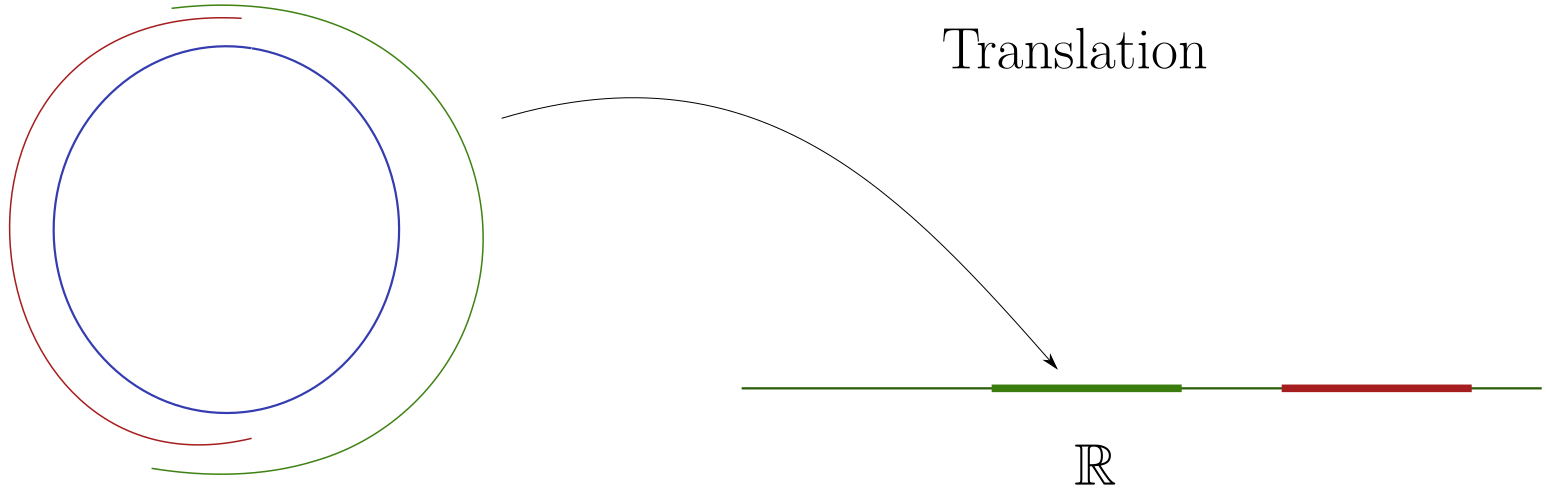Check that on a torus, $$dx_i$$ is a well-defined 1-form.

Note that there is a map $$d: \Omega^p \to \Omega^{p+1}$$ where $$\omega\mapsto d \omega$$.

$$d$$ is not a map of $${\mathcal{O}}{\hbox{-}}$$modules: $$d(f\cdot \omega) = f\cdot \omega + {\color{red} df \wedge \omega}$$, where the latter is a correction term. In particular, it is not a map of vector bundles, but is a map of sheaves of abelian groups since $$d ( \omega_1 + \omega_2) = d( \omega_1 ) + d ( \omega_2)$$, making $$d$$ a sheaf morphism.

Let $$X \in {\mathsf{Mfd}}_{\mathbb{C}}$$, we’ll use the fact that $$TX$$ is complex-linear and thus a $${\mathbb{C}}{\hbox{-}}$$vector bundle.Note that $$\Omega^p$$ for complex manifolds is $$\bigwedge^p T {}^{ \vee }$$, and so if we want to view $$X \in {\mathsf{Mfd}}_{\mathbb{R}}$$ we’ll write $$X_{{\mathbb{R}}}$$. $$TX_{\mathbb{R}}$$ is then a real vector bundle of rank $$2n$$.

$$\Omega^p$$ will denote holomorphic $$p{\hbox{-}}$$forms, i.e. local expressions of the form \begin{align*} \sum f_I(z_1, \cdots, z_n) \bigwedge dz_I .\end{align*} For example, $$e^zdz\in \Omega^1({\mathbb{C}})$$ but $$z\mkern 1.5mu\overline{\mkern-1.5muz\mkern-1.5mu}\mkern 1.5mu dz$$ is not, where $$dz = dx + idy$$. We’ll use a different notation when we allow the $$f_I$$ to just be smooth: $$A^{p, 0}$$, the sheaf of $$(p, 0){\hbox{-}}$$forms. Then $$z\mkern 1.5mu\overline{\mkern-1.5muz\mkern-1.5mu}\mkern 1.5mu dz\in A^{1, 0}$$.

Note that $$T {}^{ \vee }X_{\mathbb{R}}\otimes _{\mathbb{C}}= A^{1, 0} \oplus A^{0, 1}$$ since there is a unique decomposition $$\omega = fdz + gd\mkern 1.5mu\overline{\mkern-1.5muz\mkern-1.5mu}\mkern 1.5mu$$ where $$f,g$$ are smooth. Then $$\Omega^d X_{\mathbb{R}}\otimes_{\mathbb{R}}{\mathbb{C}}= \bigoplus _{p+q=d} A^{p, q}$$. Note that $$\Omega_{{\mathsf{sm}}}^p \neq A^{p, q}$$ and these are really quite different: the former are more like holomorphic bundles, and the latter smooth. Moreover $$\dim \Omega^p(X) < \infty$$, whereas $$\Omega_{{\mathsf{sm}}}^1$$ is infinite-dimensional.

# 6 Principal $$G{\hbox{-}}$$Bundles and Connections (Monday, January 25)

Let $$G$$ be a (possibly disconnected) Lie group. Then a principal $$G{\hbox{-}}$$bundle $$\pi:P\to X$$ is a space admitting local trivializations $$h_u: \pi ^{-1} (U) \to G \times U$$ such that the transition functions are given by left multiplication by a continuous function $$t_{UV}: U \cap V \to G$$.Setup: we’ll consider $$TX$$ for $$X\in {\mathsf{Mfd}}_{\operatorname{Sm}}$$, and let $$g$$ be a metric on the tangent bundle given by \begin{align*} g_p: T_pX^{\otimes 2} \to {\mathbb{R}} ,\end{align*} a symmetric bilinear form with $$g_p(u, v) \geq 0$$ with equality if and only if $$v=0$$.

Define $$\mathop{\mathrm{Frame}}_p(X) \mathrel{\vcenter{:}}=\left\{{\text{bases of } T_p X}\right\}$$, and $$\mathop{\mathrm{Frame}}(X) \mathrel{\vcenter{:}}=\displaystyle\bigcup_{p\in X} \mathop{\mathrm{Frame}}_p(X)$$.

More generally, $$\mathop{\mathrm{Frame}}(\mathcal{E})$$ can be defined for any vector bundle $$\mathcal{E}$$, so $$\mathop{\mathrm{Frame}}(X) \mathrel{\vcenter{:}}=\mathop{\mathrm{Frame}}(TX)$$. Note that $$\mathop{\mathrm{Frame}}(X)$$ is a principal $$\operatorname{GL}_n({\mathbb{R}}){\hbox{-}}$$bundle where $$n\mathrel{\vcenter{:}}=\operatorname{rank}(\mathcal{E})$$. This follows from the fact that the transition functions are fiberwise in $$\operatorname{GL}_n({\mathbb{R}})$$, so the transition functions are given by left-multiplication by matrices.

A principal $$G{\hbox{-}}$$bundle admits a $$G{\hbox{-}}$$action where $$G$$ acts by right multiplication: \begin{align*} P \times G \to P \\ ( (g, x), h) \mapsto (gh, x) .\end{align*} This is necessary for compatibility on overlaps. Key point: the actions of left and right multiplication commute.

The orthogonal frame bundle of a vector bundle $$\mathcal{E}$$ equipped with a metric $$g$$ is defined as $$\mathop{\mathrm{OFrame}}_p(\mathcal{E}) \mathrel{\vcenter{:}}=\left\{{\text{orthonormal bases of } \mathcal{E}_p}\right\}$$, also written $$O_r({\mathbb{R}})$$ where $$r \mathrel{\vcenter{:}}=\operatorname{rank}( \mathcal{E})$$.

The fibers $$P_x \to \left\{{x}\right\}$$ of a principal $$G{\hbox{-}}$$bundle are naturally torsors over $$G$$, i.e. a set with a free transitive $$G{\hbox{-}}$$action.

Let $$\mathcal{E}\to X$$ be a complex vector bundle. Then a Hermitian metric is a hermitian form on every fiber, i.e.  \begin{align*} h_p: \mathcal{E}_p \times\overline{\mathcal{E}_p } \to {\mathbb{C}} .\end{align*} where $$h_p(v, \mkern 1.5mu\overline{\mkern-1.5muv\mkern-1.5mu}\mkern 1.5mu ) \geq 0$$ with equality if and only if $$v=0$$. Here we define $$\overline{\mathcal{E}_p}$$ as the fiber of the complex vector bundle $$\overline{\mathcal{E}}$$ whose transition functions are given by the complex conjugates of those from $$\mathcal{E}$$.

Note that $$\mathcal{E}, \overline{\mathcal{E}}$$ are genuinely different as complex bundles. There is a conjugate-linear map given by conjugation, i.e. $$L(cv) = \mkern 1.5mu\overline{\mkern-1.5muc\mkern-1.5mu}\mkern 1.5mu L(v)$$, where the canonical example is \begin{align*} {\mathbb{C}}^n &\to {\mathbb{C}}^n \\ (z_1, \cdots, z_n) &\mapsto (\mkern 1.5mu\overline{\mkern-1.5muz_1\mkern-1.5mu}\mkern 1.5mu, \cdots, \mkern 1.5mu\overline{\mkern-1.5muz_n\mkern-1.5mu}\mkern 1.5mu) .\end{align*}

We define the unitary frame bundle $$\mathop{\mathrm{UFrame}}(\mathcal{E}) \mathrel{\vcenter{:}}=\displaystyle\bigcup_p \mathop{\mathrm{UFrame}}(\mathcal{E})_p$$, where at each point this is given by the set of orthogonal frames of $$\mathcal{E}_p$$ given by $$(e_1, \cdots, e_n)$$ where $$h(e_i , \mkern 1.5mu\overline{\mkern-1.5mue_j\mkern-1.5mu}\mkern 1.5mu ) = \delta_{ij}$$.

This is a principal $$G{\hbox{-}}$$bundle for $$G = U_r({\mathbb{C}})$$, the invertible matrices $$A_{/{\mathbb{C}}}$$ satisfy $$A \overline{A}^t = \operatorname{id}$$.

For $$G={\mathbb{Z}}/2{\mathbb{Z}}$$ and $$X= S^1$$, the Möbius band is a principal $$G{\hbox{-}}$$bundle:For $$G={\mathbb{Z}}/2{\mathbb{Z}}$$, for any (possibly non-oriented) manifold $$X$$ there is an orientation principal bundle $$P$$ which is locally a set of orientations on $$U$$, i.e.  \begin{align*} P\mathrel{\vcenter{:}}=\left\{{(x, O) {~\mathrel{\Big|}~}x\in X,\, O \text{ is an orientation of }T_p X}\right\} .\end{align*} Note that $$P$$ is an oriented manifold, $$P\to X$$ is a local isomorphism, and has a canonical orientation. (?) This can also be written as $$P = \mathop{\mathrm{Frame}}(X) / \operatorname{GL}_n^+({\mathbb{R}})$$, since an orientation can be specified by a choice of $$n$$ linearly independent vectors where we identify any two sets that differ by a matrix of positive determinant.

Let $$P\to X$$ be a principal $$G{\hbox{-}}$$bundle and let $$G\to \operatorname{GL}(V)$$ be a continuous representation. The associated bundle is defined as \begin{align*} P\times_G V = \left\{{(p, v){~\mathrel{\Big|}~}p\in P,\, v\in V}\right\} / \sim && \text{where } (p, v) \sim (pg, g ^{-1} v) ,\end{align*} which is well-defined since there is a right action on the first component and a left action on the second.

Note that $$\mathop{\mathrm{Frame}}(\mathcal{E})$$ is a $$\operatorname{GL}_r({\mathbb{R}}){\hbox{-}}$$bundle and the map $$\operatorname{GL}_r({\mathbb{R}}) \xrightarrow{\operatorname{id}} \operatorname{GL}({\mathbb{R}}^r)$$ is a representation. At every fiber, we have $$G \times_G V = (p, v)/\sim$$ where there is a unique representative of this equivalence class given by $$(e, pv)$$. So $$P\times_G V_p \to \left\{{p}\right\} \cong V_x$$.

Show that $$\mathop{\mathrm{Frame}}(\mathcal{E}) \times_{\operatorname{GL}_r({\mathbb{R}})} {\mathbb{R}}^r \cong \mathcal{E}$$. This follows from the fact that the transition functions of $$P \times_G V$$ are given by left multiplication of $$t_{UV}: U \cap V \to G$$, and so by the equivalence relation, $$\operatorname{im}t_{UV} \in \operatorname{GL}(V)$$.

Suppose that $$M^3$$ is an oriented Riemannian 3-manifold. Them $$TM\to \mathop{\mathrm{Frame}}(M)$$ which is a principal $${\operatorname{SO}}(3){\hbox{-}}$$bundle. The universal cover is the double cover $${\operatorname{SU}}(2) \to {\operatorname{SO}}(3)$$, so can the transition functions be lifted? This shows up for spin structures, and we can get a $${\mathbb{C}}^2$$ bundle out of this.

# 7 Wednesday, January 27

## 7.1 Bundles and Connections

Let $$\mathcal{E}\to X$$ be a vector bundle, then a connection on $$\mathcal{E}$$ is a map of sheaves of abelian groups \begin{align*} \nabla: \mathcal{E}\to \mathcal{E}\otimes\Omega^1_X \end{align*} satisfying the Leibniz rule: \begin{align*} \nabla (fs) = f \nabla s + s\otimes ds \end{align*} for all opens $$U$$ with $$f\in {\mathcal{O}}(U)$$ and $$s\in \mathcal{E}(U)$$. Note that this works in the category of complex manifolds, in which case $$\nabla$$ is referred to as a holomorphic connection.

A connection $$\nabla$$ induces a map \begin{align*} \tilde{\nabla}: \mathcal{E}\otimes\Omega^p &\to \mathcal{E}\otimes\Omega^{p+1} \\ s \otimes \omega &\mapsto \nabla s \wedge w + s\otimes d \omega .\end{align*} where $$\wedge: \Omega^p \otimes\Omega^1 \to \Omega^{p+1}$$. The standard example is \begin{align*} d: {\mathcal{O}}&\to \Omega^1 \\ f &\mapsto df .\end{align*} where the induced map is the usual de Rham differential.

Prove that the curvature of $$\nabla$$, i.e. the map \begin{align*} F_{\nabla} \mathrel{\vcenter{:}}=\nabla \circ \nabla: \mathcal{E}\to \mathcal{E}\otimes\Omega^2 \end{align*} is $${\mathcal{O}}{\hbox{-}}$$linear, so $$F_{\nabla}(fs) = f\nabla \circ \nabla(s)$$. Use the fact that $$\nabla s \in \mathcal{E}\otimes\Omega^1$$ and $$\omega \in \Omega^p$$ and so $$\nabla s \otimes \omega \in \mathcal{E} \Omega^1 \otimes \Omega^p$$ and thus reassociating the tensor product yields $$\nabla s \wedge \omega \in \mathcal{E}\otimes\Omega^{p+1}$$.

Why is this called a connection?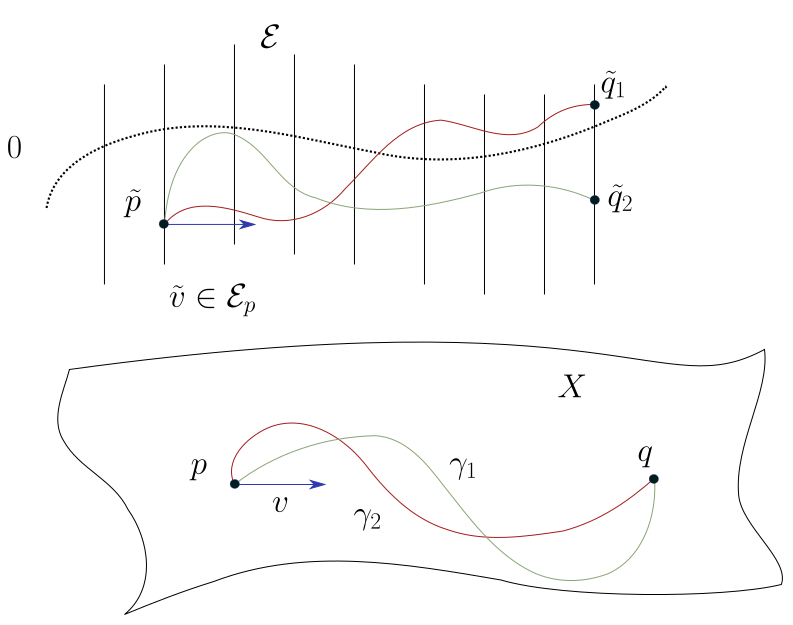This gives us a way to transport $$v\in \mathcal{E}_p$$ over a path $$\gamma$$ in the base, and $$\nabla$$ provides a differential equation (a flow equation) to solve that lifts this path. Solving this is referred to as parallel transport. This works by pairing $$\gamma'(t) \in T_{ \gamma(t) } X$$ with $$\Omega^1$$, yielding $$\nabla s = ( \gamma'(t)) = s( \gamma(t))$$ which are sections of $$\gamma$$.

Note that taking a different path yields an endpoint in the same fiber but potentially at a different point, and $$F_\nabla = 0$$ if and only if the parallel transport from $$p$$ to $$q$$ depends only on the homotopy class of $$\gamma$$.

Note: this works for any bundle, so can become confusing in Riemannian geometry when all of the bundles taken are tangent bundles!

The Levi-Cevita connection $$\nabla^{LC}$$ on $$TX$$, which depends on a metric $$g$$. Taking $$X=S^2$$ and $$g$$ is the round metric, there is nonzero curvature: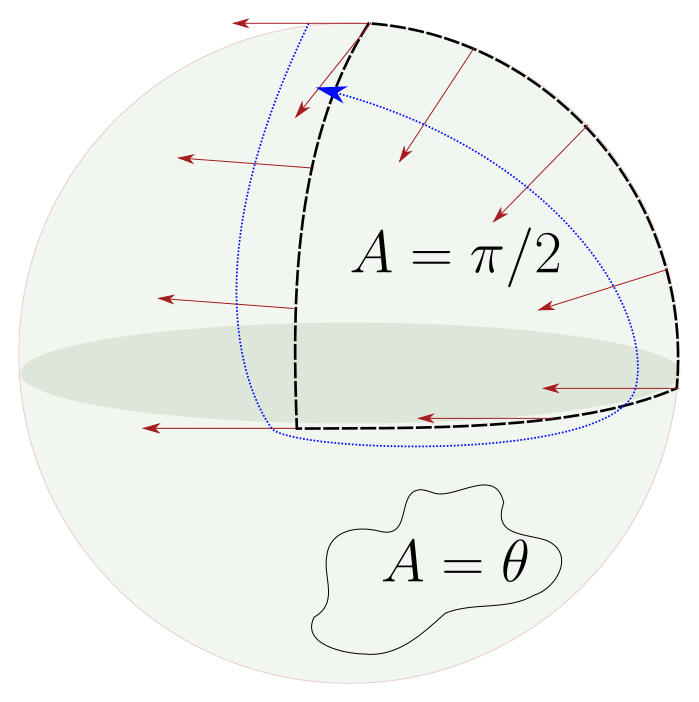In general, every such transport will be rotation by some vector, and the angle is given by the area of the enclosed region.

A connection is flat if $$F_\nabla = 0$$. A section $$s \in \mathcal{E}(U)$$ is flat if it is given by \begin{align*} L(U) \mathrel{\vcenter{:}}=\left\{{ s\in \mathcal{E}(U) {~\mathrel{\Big|}~}\nabla s = 0}\right\} .\end{align*}

Show that if $$\nabla$$ is flat then $$L$$ is a local system: a sheaf that assigns to any sufficiently small open set a vector space of fixed dimension. An example is the constant sheaf $$\underline{{\mathbb{C}}^d}$$. Furthermore $${\operatorname{rank}}(L) = {\operatorname{rank}}(\mathcal{E})$$.

Given a local system, we can construct a vector bundle whose transition functions are the same as those of the local system, e.g. for vector bundles this is a fixed matrix, and in general these will be constant transition functions. Equivalently, we can take $$L\otimes_{\mathbb{R}}{\mathcal{O}}$$, and $$L\otimes 1$$ form flat sections of a connection.

## 7.2 Sheaf Cohomology

Let $$\mathcal{F}$$ be a sheaf of abelian groups on a topological space $$X$$, and let $$\mathfrak{U} \mathrel{\vcenter{:}}=\left\{{U_i}\right\} \rightrightarrows X$$ be an open cover of $$X$$. Let $$U_{i_1, \cdots, i_p} \mathrel{\vcenter{:}}= U_{i_1} \cap U_{i_2} \cap\cdots \cap U_{i_p}$$. Then the Čech Complex is defined as \begin{align*} C_{\mathfrak{U}}^p(X, \mathcal{F}) \mathrel{\vcenter{:}}=\prod_{i_1 < \cdots < i_p} \mathcal{F}(U_{i_1, \cdots, i_p}) \end{align*} with a differential \begin{align*} {{\partial}}^p: C_{\mathfrak{U}}^p(X, \mathcal{F}) &\to C_{\mathfrak{U}}^{p+1}(X \mathcal{F}) \\ \sigma &\mapsto ({{\partial}}\sigma)_{i_0, \cdots, i_p} \mathrel{\vcenter{:}}=\prod_j (-1)^j { \left.{{\sigma_{i_0, \cdots, \widehat{i_j}, \cdots, i_p}}} \right|_{{U_{i_0, \cdots, i_p}}} } \end{align*} where we’ve defined this just on one given term in the product, i.e. a $$p{\hbox{-}}$$fold intersection.

Check that $${{\partial}}^2 = 0$$.

The Čech cohomology $$H^p_{\mathfrak{U}}(X, \mathcal{F})$$ with respect to the cover $$\mathfrak{U}$$ is defined as $$\ker {{\partial}}^p/\operatorname{im}{{\partial}}^{p-1}$$. It is a difficult theorem, but we write $$H^p(X, \mathcal{F})$$ for the Čech cohomology for any sufficiently refined open cover when $$X$$ is assumed paracompact.

Consider $$S^1$$ and the constant sheaf $$\underline{{\mathbb{Z}}}$$: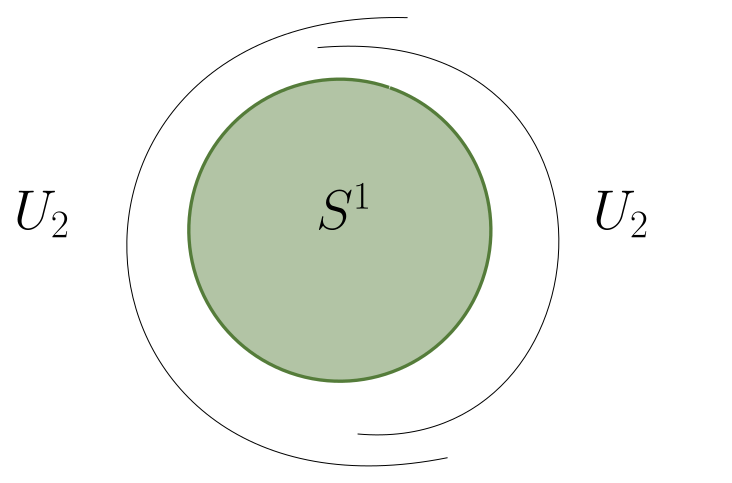ere we have \begin{align*} C^0(S^1, \underline{{\mathbb{Z}}}) = \underline{{\mathbb{Z}}}(U_1) \oplus \underline{{\mathbb{Z}}}(U_2) = \underline{{\mathbb{Z}}} \oplus \underline{{\mathbb{Z}}} ,\end{align*} and \begin{align*} C^1(S^1, {\mathbb{Z}}) = \bigoplus_{\substack{ \text{double} \\ \text{intersections}} } \underline{{\mathbb{Z}}}(U_{ij}) \underline{{\mathbb{Z}}}(U_{12}) = \underline{{\mathbb{Z}}}(U_1 \cap U_{2}) = \underline{{\mathbb{Z}}} \oplus \underline{{\mathbb{Z}}} .\end{align*} We then get \begin{align*} C^0(S^1, \underline{{\mathbb{Z}}}) &\xrightarrow{{{\partial}}} C^1(S^1, \underline{{\mathbb{Z}}}) \\ {\mathbb{Z}}\oplus {\mathbb{Z}}&\to {\mathbb{Z}}\oplus {\mathbb{Z}}\\ (a, b) &\mapsto (a-b, a-b) ,\end{align*}

Which yields $$H^*(S^1, \underline{{\mathbb{Z}}}) = [{\mathbb{Z}}, {\mathbb{Z}}, 0, \cdots]$$.

# 8 Sheaf Cohomology (Friday, January 29)

Last time: we defined the Čech complex $$C_{\mathfrak{U} }^p(X, \mathcal{F} ) \mathrel{\vcenter{:}}=\prod_{i_1, \cdots, i_p} \mathcal{F} (U_{i_1} \cap\cdots \cap U_{i_p})$$ for $$\mathfrak{U}\mathrel{\vcenter{:}}=\left\{{U_i}\right\}$$ is an open cover of $$X$$ and $$F$$ is a sheaf of abelian groups.

If $$\mathfrak{U}$$ is a sufficiently fine cover then $$H^p_{\mathfrak{U}}(X, \mathcal{F})$$ is independent of $$\mathfrak{U}$$, and we call this $$H^p(X; \mathcal{F})$$.

Recall that we computed $$H^p(S^1, \underline{{\mathbb{Z}}} = [{\mathbb{Z}}, {\mathbb{Z}}, 0, \cdots]$$.

Let $$X$$ be a paracompact and locally contractible topological space. Then $$H^p(X, \underline{{\mathbb{Z}}}) \cong H^p_{{\operatorname{Sing}}}(X, \underline{{\mathbb{Z}}})$$. This will also hold more generally with $$\underline{{\mathbb{Z}}}$$ replaced by $$\underline{A}$$ for any $$A\in {\mathsf{Ab}}$$.

We say $$\mathcal{F}$$ is acyclic on $$X$$ if $$H^{> 0 }(X; \mathcal{F}) = 0$$.

How to visualize when $$H^1(X; \mathcal{F}) = 0$$: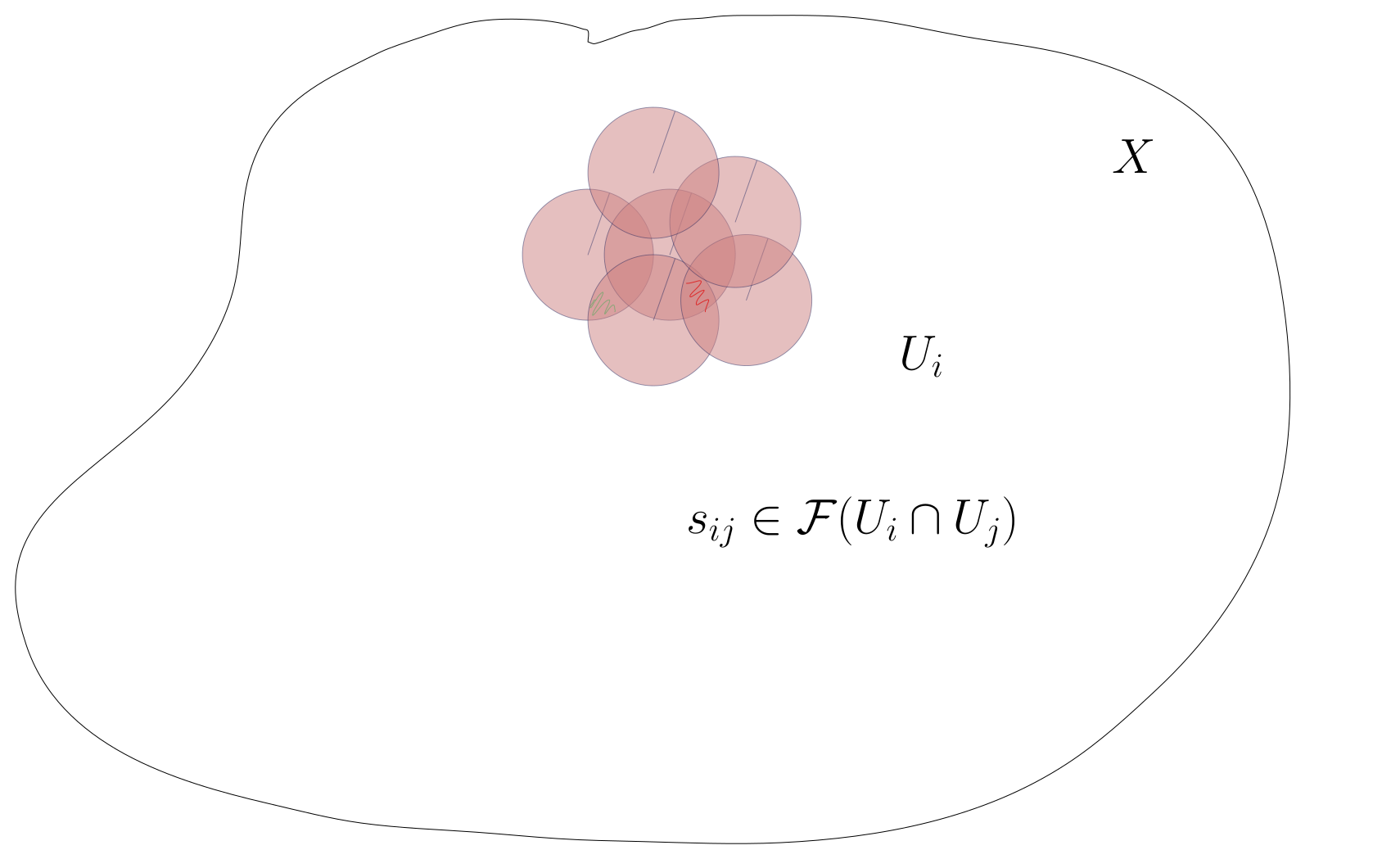On the intersections, we have $$\operatorname{im}{{\partial}}^0 = \left\{{ (s_{i} - s_{j})_{ij} {~\mathrel{\Big|}~}s_i \in \mathcal{F}(U_i)}\right\}$$, which are cocycles. We have $$C^1(X; \mathcal{F})$$ are collections of sections of $$\mathcal{F}$$ on every double overlap. We can check that $$\ker {{\partial}}^1 = \left\{{ (s_{ij}) {~\mathrel{\Big|}~}s_{ij} - s_{ik} + s_{jk} = 0}\right\}$$, which is the cocycle condition. From the exercise from last class, $${{\partial}}^2 = 0$$.

Let $$X$$ be a paracompact Hausdorff space and let \begin{align*} 0 \to \mathcal{F}_1 \xrightarrow{\varphi} \mathcal{F}_2 \to \mathcal{F}_3 \to 0 \end{align*} be a SES of sheaves of abelian groups, i.e. $$\mathcal{F}_3 = \operatorname{coker}(\varphi)$$ and $$\varphi$$ is injective. Then there is a LES in cohomology:For $$X$$ a manifold, we can define a map and its cokernel sheaf:

\begin{align*} 0 \to \underline{{\mathbb{Z}}} \xrightarrow{\cdot 2} \underline{{\mathbb{Z}}} \to \underline{{\mathbb{Z}}/2{\mathbb{Z}}} \to 0 .\end{align*} Using that cohomology of constant sheaves reduces to singular cohomology, we obtain a LES in homology:Suppose $$0 \to \mathcal{F}\to I_0 \xrightarrow{d_0} I_1 \xrightarrow{d_1} I_2 \xrightarrow{d_2} \cdots$$ is an exact sequence of sheaves, so on any sufficiently small set kernels equal images., and suppose $$I_n$$ is acyclic for all $$n\geq 0$$. This is referred to as an acyclic resolution. Then the homology can be computed at $$H^p(X; \mathcal{F}) = \ker (I_p(X) \to I_{p+1}(X)) / \operatorname{im}(I_{p-1}(X) \to I_p(X) )$$.

Note that locally having kernels equal images is different than satisfying this globally!

This is a formal consequence of the existence of the LES. We can split the LES into a collection of SESs of sheaves:

\begin{align*} 0 \to \mathcal{F}\to I_0 \xrightarrow{d_0} \operatorname{im}(d_0) \to 0 && \operatorname{im}(d_0) = \ker(d_1) \\ 0 \to \ker(d_1) \hookrightarrow I_1 \to I_1/\ker (d_1) = \operatorname{im}(d_1) && \operatorname{im}(d_1) = \ker(d_2) \\ .\end{align*} Note that these are all exact sheaves, and thus only true on small sets. So take the associated LESs. For the SES involving $$I_0$$, we obtain: